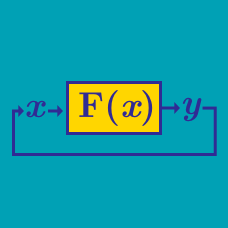Computer Science

# Memoizationbottom-up

When implementing a dynamic programming solution, computing all values in a bottom-up fashion(tabulation) is asymptotically faster than using recursion and memoization.

The following python function computes two sequences A(n) and B(n). Unfortunately, it is very slow. You're task is to memoize the two functions. What are the last three digits of the output of the program?

  1 2 3 4 5 6 7 8 9 10 11 12 13 14 def A(n): if n < 3: return 1 else: return B(n-1) + B(n-2) def B(n): if n < 3: return 2 else: return A(n-1) + B(n-2) print B(100) 

The code below is a solution to the classic LCS problem.

 1 2 3 4 5 6 7 LCS(S,n,T,m) { if (n==0 or m==0) return 0 if (S[n] == T[m]) result = 1 + LCS(S,n-1,T,m-1) else result = max( LCS(S,n-1,T,m), LCS(S,n,T,m-1) ) return result } 

It is clearly a pure recursive solution. Converting it to a top-down Dynamic Programming solution involves adding the following two lines.

A:

 1 if (A[n][m] != nill) return A[n][m] 

B:

 1 arr[n][m] = result; 

Details and Assumptions

• A is a 2D array used as the memoization table.
• nill` is what each item in the 2D array are initialized to.
×